Next: Propagator Theory Up: Propagator Methods Previous: Propagator Methods

# Green's Functions

Propagator theory is based on the Green's function method of solving inhomogeneous differential equations. We explain the method in terms of a single example.

Suppose we wish to solve Poisson's equation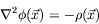(6.1)

for a known charge distribution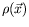, subject to some boundary conditions. It is easier to first solve the unit source'' problem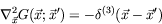(6.2)

where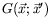is the potential at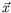due to a unit source at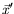. We then move this source over the charge distribution and accumulate the total potential atfrom all possible volume elements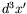: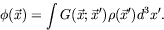(6.3)

We can check directly that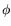is the desired solution.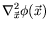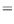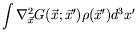(6.4)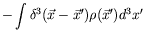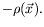In the case of the electron propagator,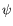appears on both sides of the solution, and so an iterative perturbation series-solution in powers of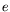is required.Next: Propagator Theory Up: Propagator Methods Previous: Propagator Methods
Douglas M. Gingrich (gingrich@ ualberta.ca)
2004-03-18